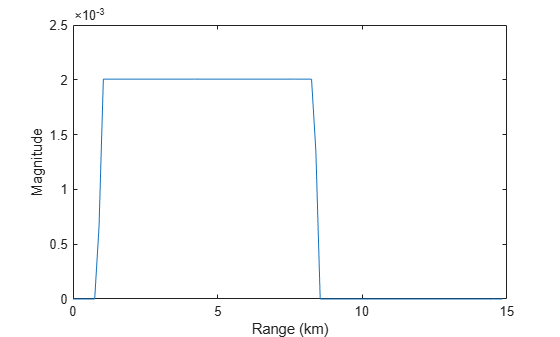## Syntax

``sig = receive(scenario)``
``[sig,info] = receive(scenario)``
``[sig,info,pids] = receive(scenario)``

## Description

````sig = receive(scenario)` returns the target echos, `sig`, received at radars in the `scenario`.```

example

````[sig,info] = receive(scenario)` also returns a structure array of configurations at each radar, `info`, of each radar when the signal is received.```

example

````[sig,info,pids] = receive(scenario)` also returns a column array of platform IDs `pids`.```

## Examples

collapse all

Obtain the signal from two platforms in the a radar scenario using a radar detection generator. Set the random number seed to insure the repeatability of the data.

```s = rng(0); scenario = radarScenario('UpdateRate',1); plat1 = platform(scenario); plat1.Trajectory.Position = [0,0,0]; plat1.Sensors = radarDataGenerator(1); plat2 = platform(scenario); plat2.Trajectory.Position = [1000,0,0]; [sig,sensorConfs] = receive(scenario)```
```sig = 1x1 cell array {1001x1 double} ```
```sensorConfs = struct with fields: IsScanDone: 0 MechanicalAngle: 0 ElectronicAngle: [2x1 double] OriginPosition: [3x1 double] Orientation: [3x3 double] ReferenceSensorIndex: 1 Sensor: [1x1 radarTransceiver] ```

Platform 1 receives echo from platform 2:

```rgrid = physconst('lightspeed')*(0:size(sig{1},1)-1)/ ... (2*sensorConfs.Sensor.Waveform.SampleRate)/1e3; plot(rgrid,abs(sig{1}),'r') xlabel('Range (km)') ylabel('Magnitude')```Obtain the signal from two platforms in a radar scenario using a radarTransceiver. Set the seed in the random number generator to obtain repeatable data.

```s = rng(0); scenario = radarScenario('UpdateRate', 1); plat1 = platform(scenario); plat1.Trajectory.Position = [0,0,0]; plat1.Sensors = radarTransceiver; plat2 = platform(scenario); plat2.Trajectory.Position = [1000,0,0];```

`[sig,sensorConfs,sensorConfPIDs] = receive(scenario)`
```sig = 1x1 cell array {100x2 double} ```
```sensorConfs = struct with fields: IsScanDone: 0 MechanicalAngle: 0 ElectronicAngle: [2x1 double] OriginPosition: [3x1 double] Orientation: [3x3 double] ReferenceSensorIndex: 0 Sensor: [1x1 radarTransceiver] ```
```sensorConfPIDs = 1 ```

```rgrid = physconst('lightspeed')*(0:size(sig{1},1)-1)/ ... (2*sensorConfs.Sensor.Waveform.SampleRate)/1e3; plot(rgrid,abs(sig{1})) xlabel('Range (km)') ylabel('Magnitude')```Return the random number generator to its previous state

`rng(s)`

## Input Arguments

collapse all

Radar scenario, specified as a `radarScenario` object.

## Output Arguments

collapse all

Complex Number Support: Yes

Radar configurations, returned as a structure array. Each structure contains the following fields:

• `IsScanDone` –– Whether one period of mechanical scan is done

• `MechanicalAngle` –– Current antenna pointing angle due to mechanical scan

• `Origin` –– Radar location in the platform coordinate system

• `Orientation` –– Radar orientation axes in the platform coordinate system

Data Types: `struct`

Platform IDs on which radars are mounted, returned as a column vector of real values.

Data Types: `double`

## Version History

Introduced in R2021a